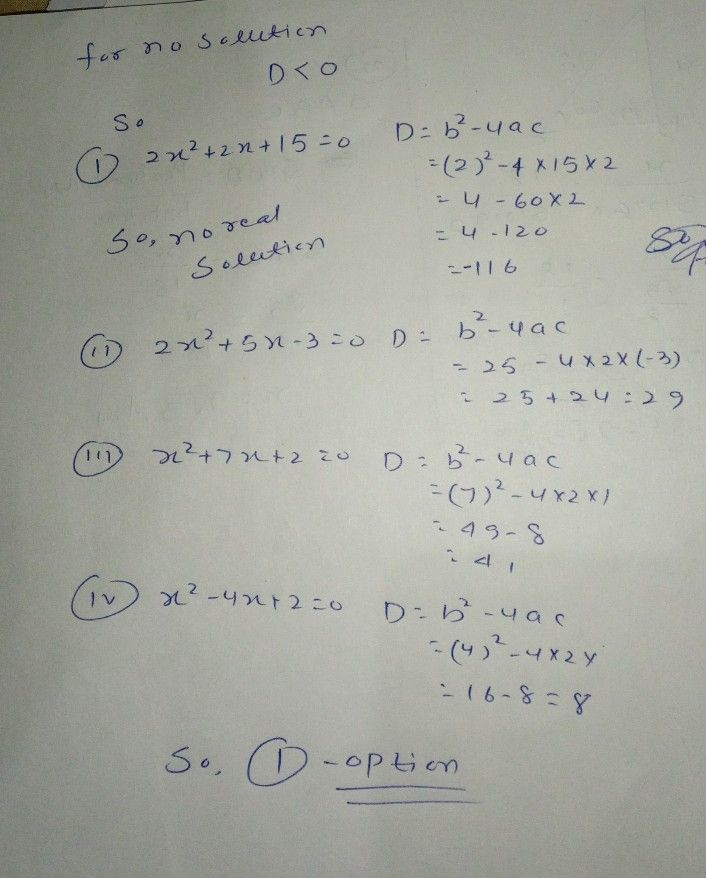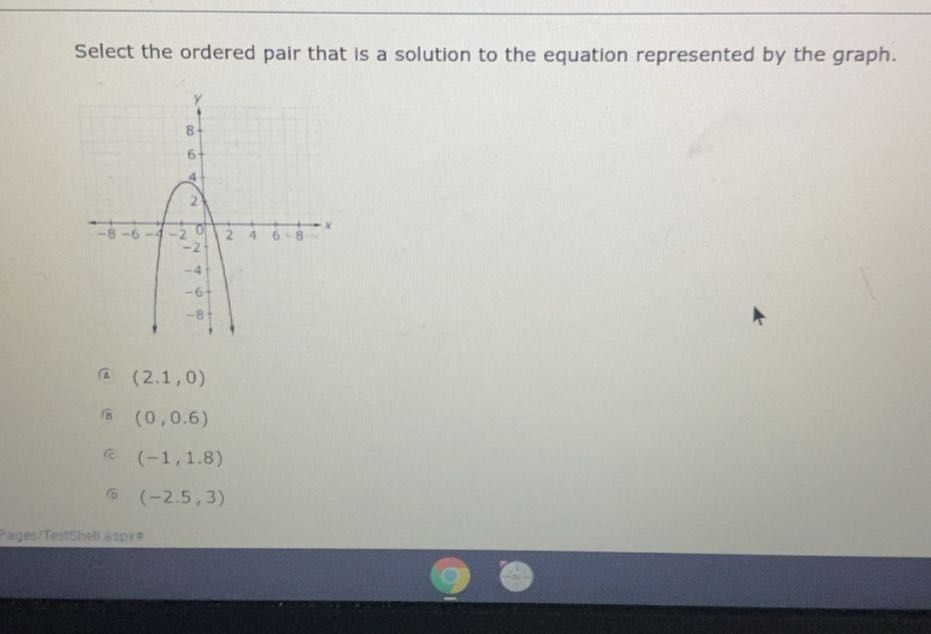Symbol
ProblemWhich equation has no real solutions? A $2x^{2}+2x+15=0$ (B $2x^{2}+5x-3=0$ © x2+7x+2=0 (D) x2-4x+2=0
10th-13th grade
Algebra
SolutionQanda teacher - Suraj_nitpStudent
Thank u so much